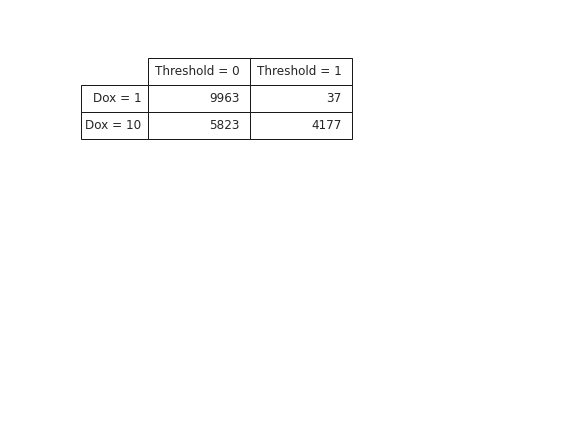# cytoflow.views.table¶

class cytoflow.views.table.TableView[source]

“Plot” a tabular view of a statistic. Mostly useful for GUIs. Each level of the statistic’s index must be used in row_facet, column_facet, subrow_facet, or subcolumn_facet. This module can’t “plot” a statistic with more than four index levels unless subset is set and that results in extra levels being dropped.

statistic

The name of the statistic to plot. Must be a key in the statistics attribute of the Experiment being plotted. Each level of the statistic’s index must be used in row_facet, column_facet, subrow_facet, or subcolumn_facet.

Type: (str, str)
row_facet, column_facet

The statistic facets to be used as row and column headers.

Type: str
subrow_facet, subcolumn_facet

The statistic facets to be used as subrow and subcolumn headers.

Type: str
subset

A Python expression used to select a subset of the statistic to plot.

Type: str

Examples

Make a little data set.

>>> import cytoflow as flow
>>> import_op = flow.ImportOp()
>>> import_op.tubes = [flow.Tube(file = "Plate01/RFP_Well_A3.fcs",
...                              conditions = {'Dox' : 10.0}),
...                    flow.Tube(file = "Plate01/CFP_Well_A4.fcs",
...                              conditions = {'Dox' : 1.0})]
>>> import_op.conditions = {'Dox' : 'float'}
>>> ex = import_op.apply()


Add a threshold gate

>>> ex2 = flow.ThresholdOp(name = 'Threshold',
...                        channel = 'Y2-A',
...                        threshold = 2000).apply(ex)


>>> ex3 = flow.ChannelStatisticOp(name = "ByDox",
...                               channel = "Y2-A",
...                               by = ['Dox', 'Threshold'],
...                               function = len).apply(ex2)


“Plot” the table

>>> flow.TableView(statistic = ("ByDox", "len"),
...                row_facet = "Dox",
...                column_facet = "Threshold").plot(ex3)plot(experiment, plot_name=None, **kwargs)[source]

Plot a table

export(experiment, filename)[source]

Export the table to a file.